Question

Power Systems

Questions 7 to 14 pertain to the control-to-output transfer function Gyd(s) of a boost converter.This converter operates with the following element values and at the following quiescent operating point:

• Input voltage Vg = 12 V • Output voltage V = 30 V • Output power P = 60 W

• Inductance L = 120µH • Capacitance C = 100µF • Switching frequency fs = 200 kHz

You may assume that all components are ideal and neglect losses. You may assume that the load is resistive. First, solve the canonical model, with the parameters listed in Table 7.1, to find an expression for Gvd(s) of the boost converter.Second, construct the magnitude and phase asymptotes of the Bode plot of Gvd(s), using the methods described in the lectures. Semilog paper is linked to the Supplemental Materials which you can print out and use for this part. Compute all break frequencies and slopes. Then answer the questions below. Enter numerical values of frequency in Hz, rounded to the nearest 1 Hz.

7. (10 points) At what frequency do the magnitude asymptotes pass through 0 dB?Verified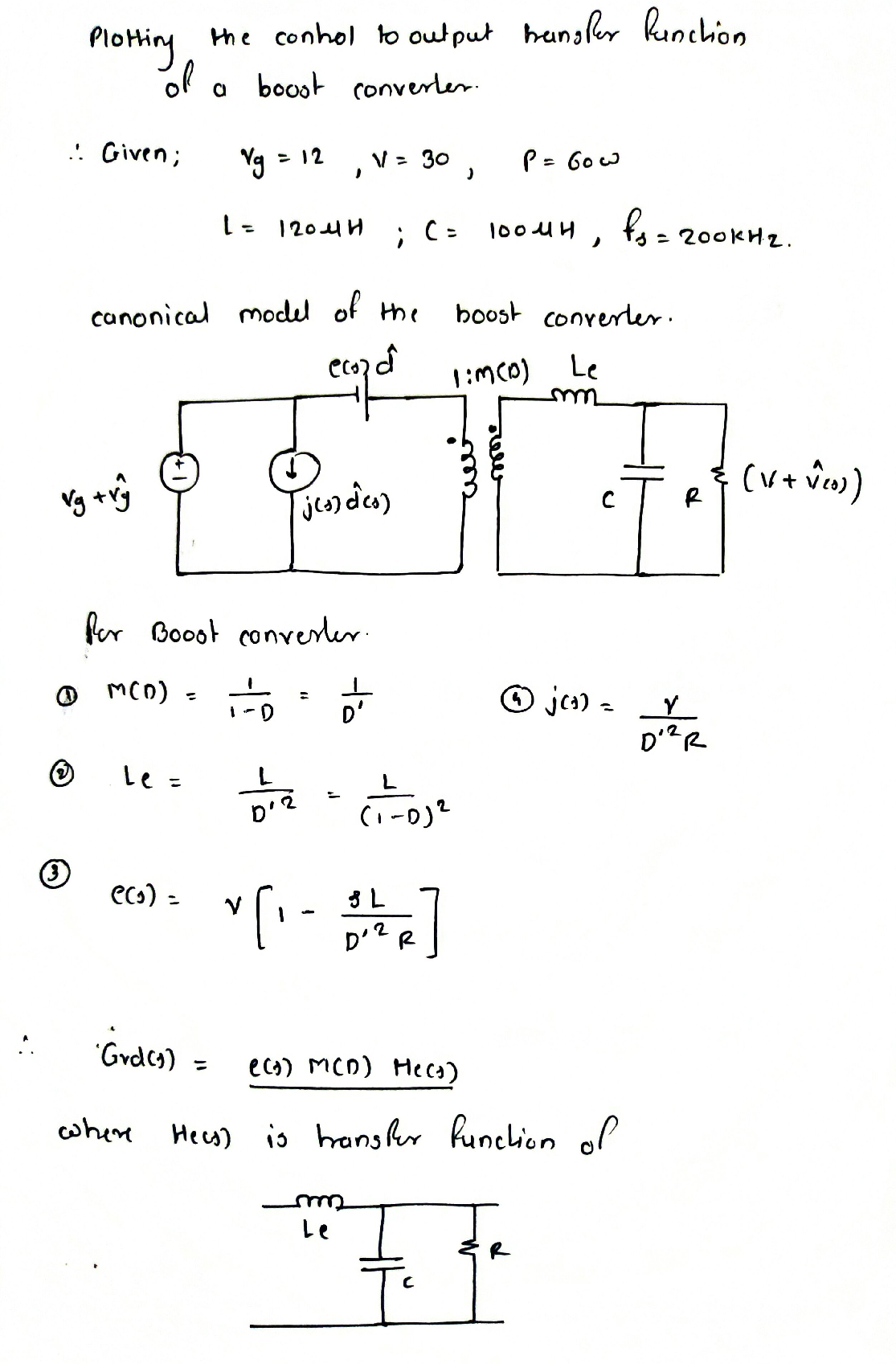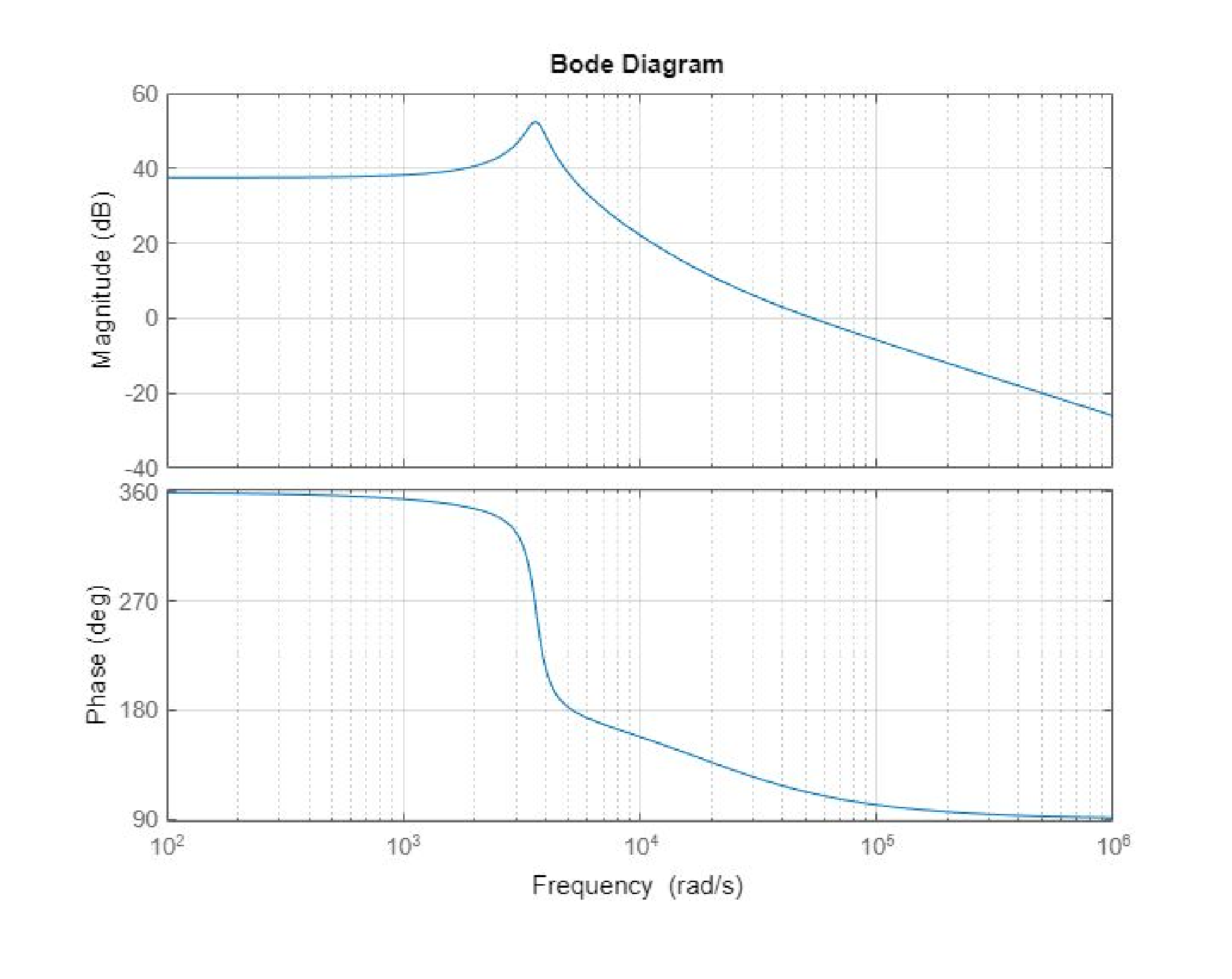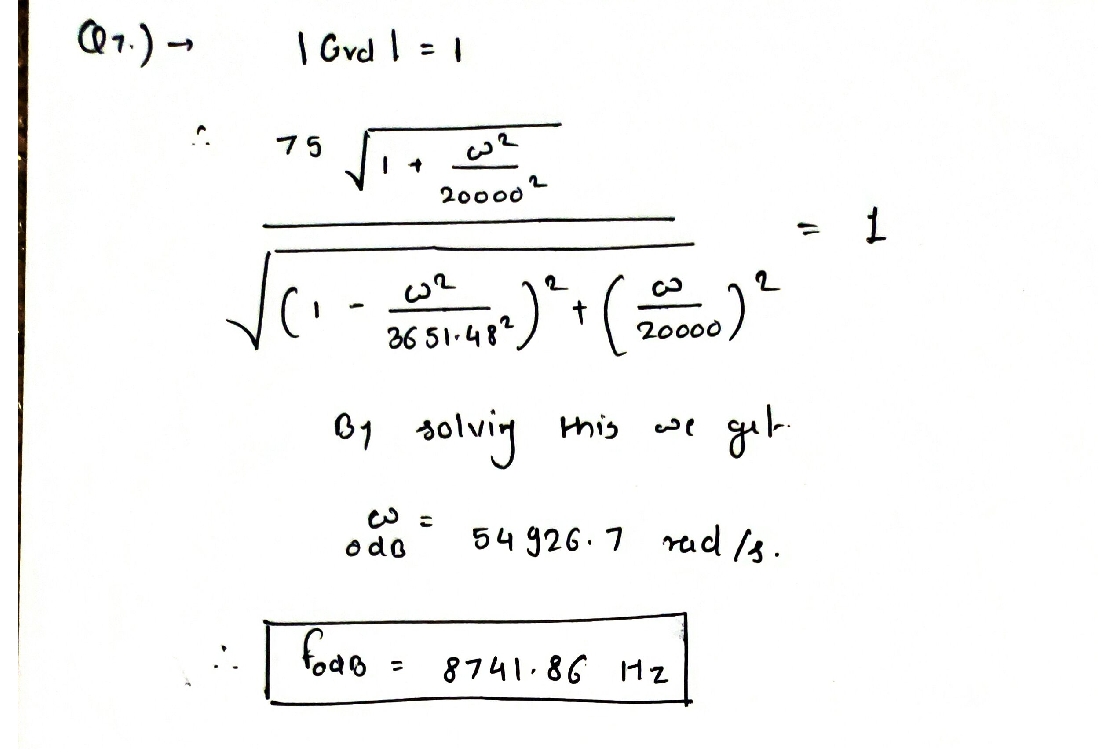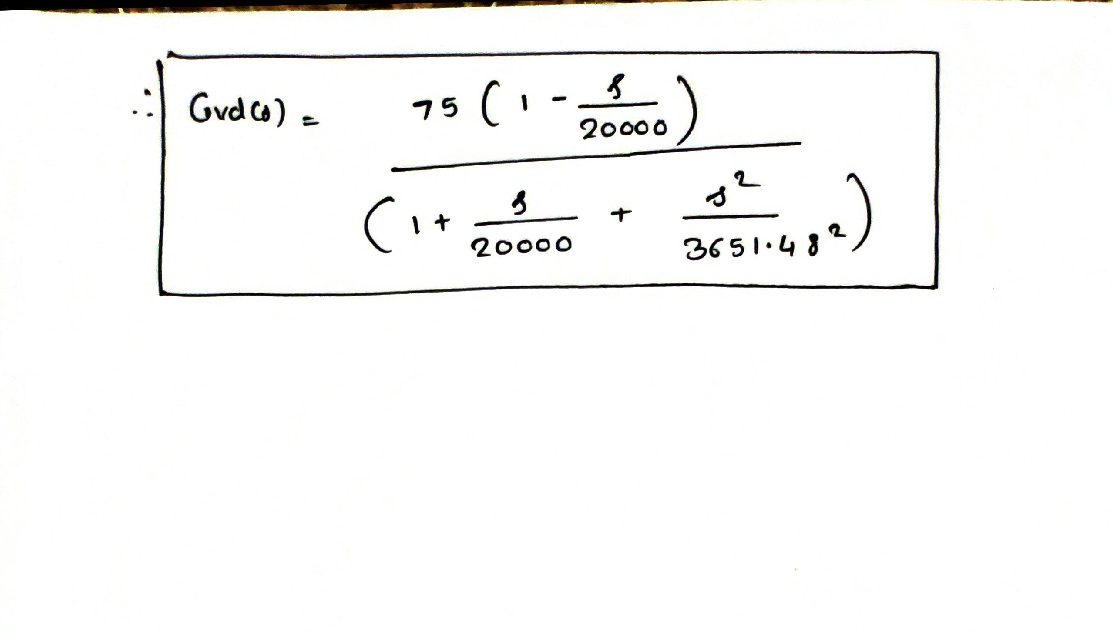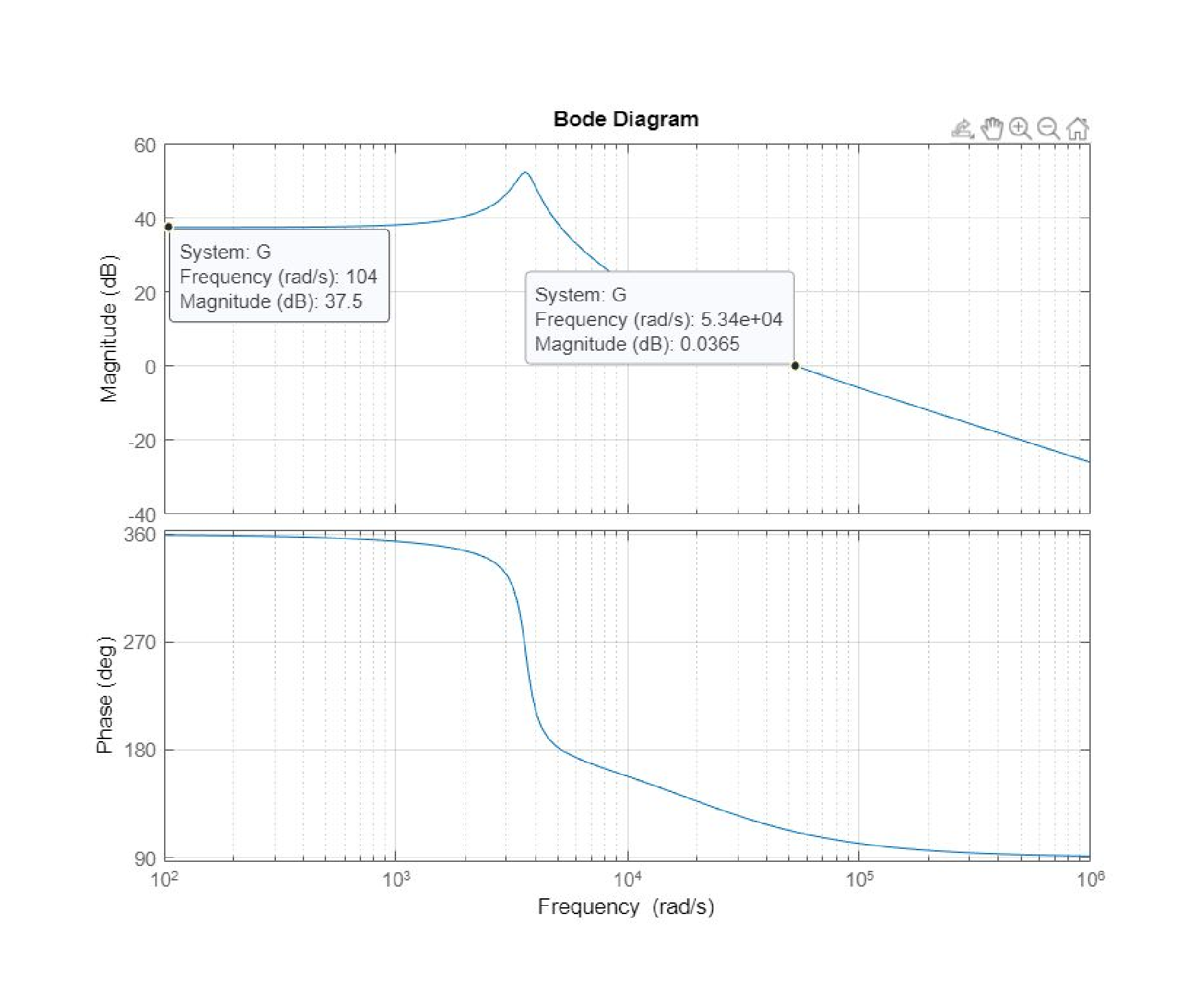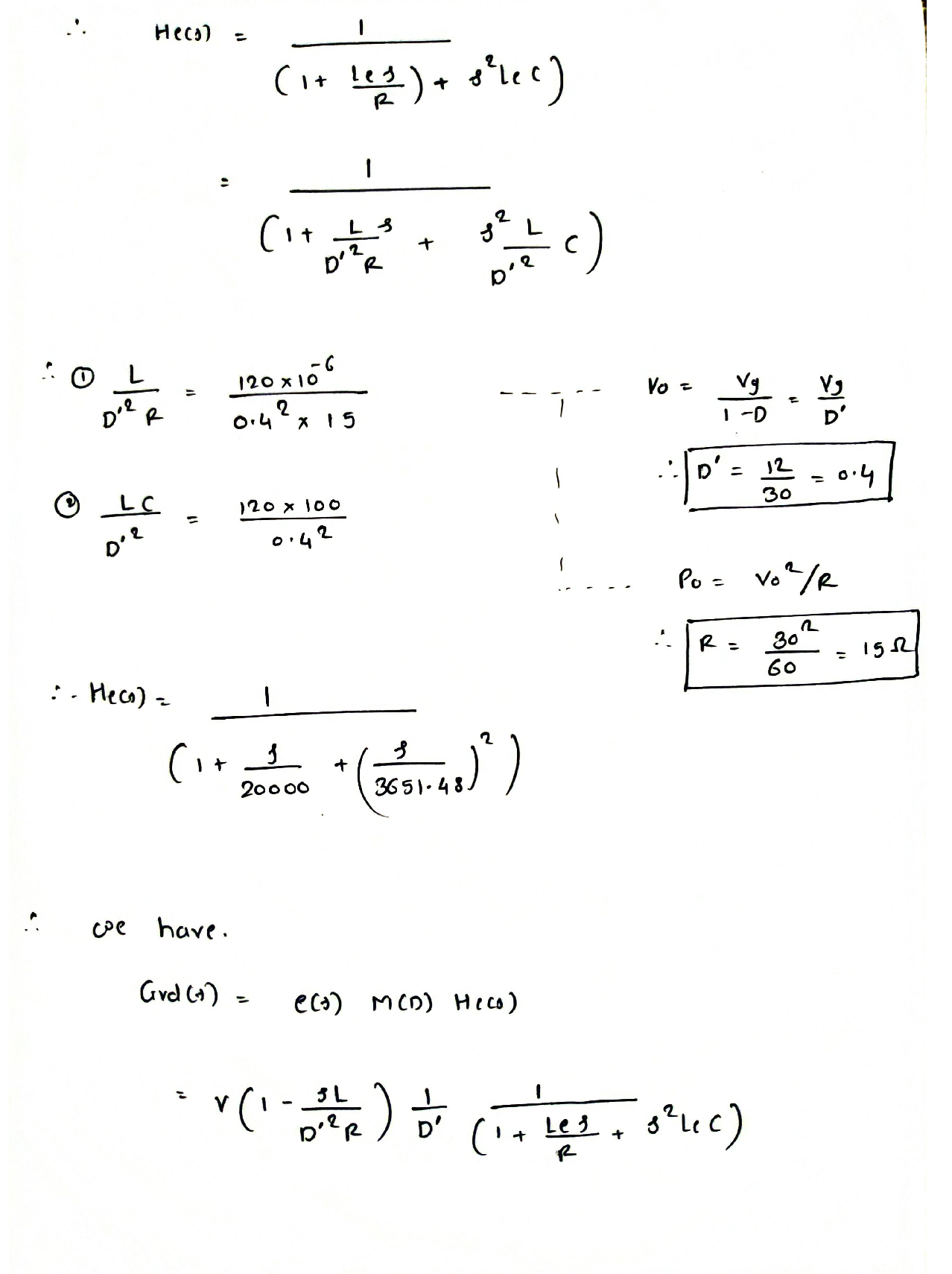### Question 45571Power Systems

State the advantages and disadvantages of using Time-of-Use (TOU) pricing strategy for EV charging.

### Question 45570Power Systems

Discuss the measures to mitigate EV load impacts by upgrading the distribution system infrastructure.

### Question 45569Power Systems

State the differences between North American distribution circuit and European distribution circuit.

### Question 45568Power Systems

Discuss the various impacts of electric vehicle load on the distribution system.

### Question 45567Power Systems

Discuss the mitigation measures for high voltage and low voltage impacts of Perpetration.

### Question 45566Power Systems

Enumerate the functions of a smart inverter.

### Question 45565Power Systems

Discuss the impact of high PV penetration on automatic voltage regulation equipment.

### Question 45564Power Systems

What is a masked load? How does it occur? Discuss its impact on the distribution circuits.

### Question 45563Power Systems

Enumerate the various impacts of high PV penetration on the distribution system.

### Question 45562Power Systems

How does the document define “high penetration PV"?

### Submit query